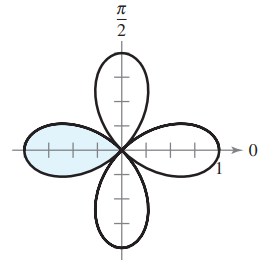×
Get Full Access to Calculus: Early Transcendental Functions - 6 Edition - Chapter 10.5 - Problem 2
Get Full Access to Calculus: Early Transcendental Functions - 6 Edition - Chapter 10.5 - Problem 2

×

# ?In Exercises 1-4, write an integral that represents the area of the shaded region of the figure. Do not evaluate the integral. $$r=\cos 2 \thISBN: 9781285774770 141 ## Solution for problem 2 Chapter 10.5 Calculus: Early Transcendental Functions | 6th Edition • Textbook Solutions • 2901 Step-by-step solutions solved by professors and subject experts • Get 24/7 help from StudySoup virtual teaching assistantsCalculus: Early Transcendental Functions | 6th Edition 4 5 1 320 Reviews 25 3 Problem 2 In Exercises 1-4, write an integral that represents the area of the shaded region of the figure. Do not evaluate the integral. \(r=\cos 2 \theta$$Text Transcription:

r = cos 2 theta

Step-by-Step Solution:

Step 1 of 5) Since ln xr and r ln x have the same derivative,ln xr = r ln x + C for some constant C. Taking x to be 1 identifies C as zero, and we’re done. You are asked to prove the Quotient Rule for logarithms, ln abx b =

Step 2 of 2

##### ISBN: 9781285774770

The answer to “?In Exercises 1-4, write an integral that represents the area of the shaded region of the figure. Do not evaluate the integral.$$r=\cos 2 \theta$$ Text Transcription:r = cos 2 theta” is broken down into a number of easy to follow steps, and 30 words. Since the solution to 2 from 10.5 chapter was answered, more than 225 students have viewed the full step-by-step answer. This full solution covers the following key subjects: . This expansive textbook survival guide covers 134 chapters, and 10738 solutions. Calculus: Early Transcendental Functions was written by and is associated to the ISBN: 9781285774770. This textbook survival guide was created for the textbook: Calculus: Early Transcendental Functions, edition: 6. The full step-by-step solution to problem: 2 from chapter: 10.5 was answered by , our top Calculus solution expert on 11/14/17, 10:53PM.

## Discover and learn what students are asking

Statistics: Informed Decisions Using Data : Tests for Independence and the Homogeneity of Proportions
?What’s in a Word? In a recent survey conducted by the Pew Research Center, a random sample of adults 18 years of age or older living in the continenta

Statistics: Informed Decisions Using Data : Inference about the Difference between Two Medians: Dependent Samples
?Effect of Aspirin on Blood Clotting Blood clotting is due to a sequence of chemical reactions. The protein thrombin initiates blood clotting by workin

Unlock Textbook Solution